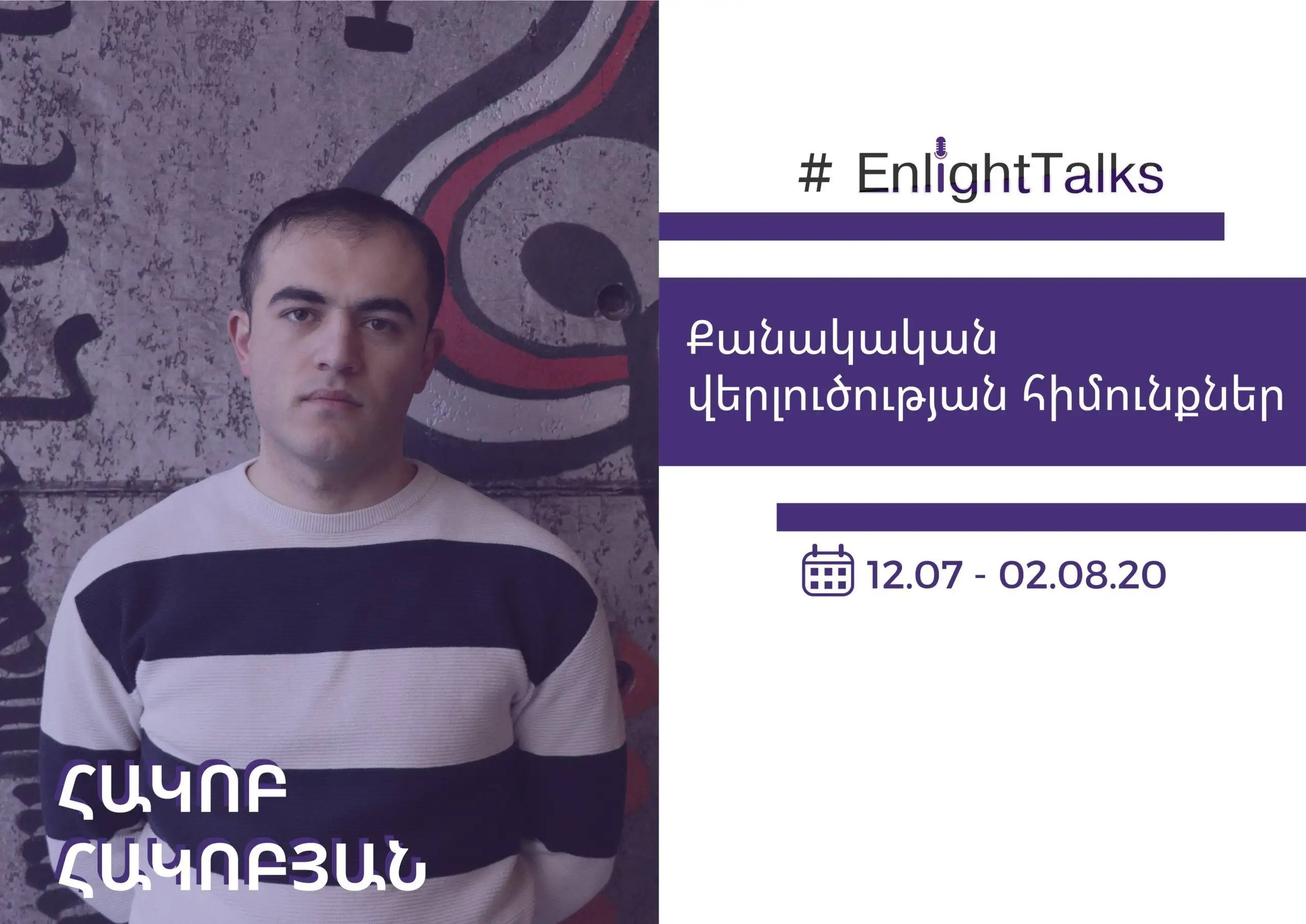# A series of discourses on “The Basics of Quantitative Analysis”#EnlightTalks series within the framework of “Enlight” three-month analytical internship program.

From July 7 to August 2, within the frameworks of analytical internship, Enlight’s analyst, economist by profession Hakob Hakobyan’s series of discourses on “Basics of quantitative analysis” was held.

During the first discourse, the speaker thoroughly introduced the types of data: panel data, longitudinal data and time series, the differences between the types of data and besides, a general introduction of probability and statistics was implemented. While speaking about time series, the speaker mentioned that for their accurate research, long time and periodically recruited data are needed. At the same time, it was mentioned that depending on the index sort, behaviour and features, the necessary period for the index assessment may be different. The speaker referred to the indexes of descriptive statistics, introduced the statistical average or the expected value, median, mode, quartiles, standard deviation or dispersion, kurtosis, and the necessity and opportunities of the use of each were introduced.

During the second discourse, the speaker introduced in detail the ways of distribution, referred to slope explaining that their positivity and negativity is a key to deciding the way of distribution and slope. Speaking about normal (Gaussian) distribution, the speaker mentioned that every distribution can be led to normal distribution due to levelling and explained the method of introduction. It was said that for introducing the correlation between 2 variables and the behaviour, covariance is used. Then for the assessment of the connection volume of those variables, the correlation was introduced. The speaker said that if the correlation is close to 1,  it is positive. If the correlation is close to -1, it is negative. It was mentioned that for the arithmetical introduction of the interaction of indexes, regression is used. The speaker introduced the regression implemented due to the method of the smallest squares and the necessary conditions or the assumptions of it. At the end of the second discourse, the speaker referred to the use of STATA and introduced some practical examples.

In order to make the third discourse more interactive, the speaker carried out discussions based on the materials assigned beforehand. As a result of the discussions, the elimination of the free term and the coefficient of linear regression equation were implemented which gave the participants of the discourse an opportunity to perceive the applied meaning of the implementation of regression analysis more vividly. Then, a regression analysis of statistical data chosen beforehand was implemented due to STATA.

At the last meeting, the speaker answered the questions of the participants, the course was summed up and an assessment of the results was carried out.

Prepared by Narine Petrosyan.

You can get acquainted with Hakob Hakobyan’s analytical articles by following this link.